Chapter 1 Class 12 Relation and Functions

Class 12
Important Questions for exams Class 12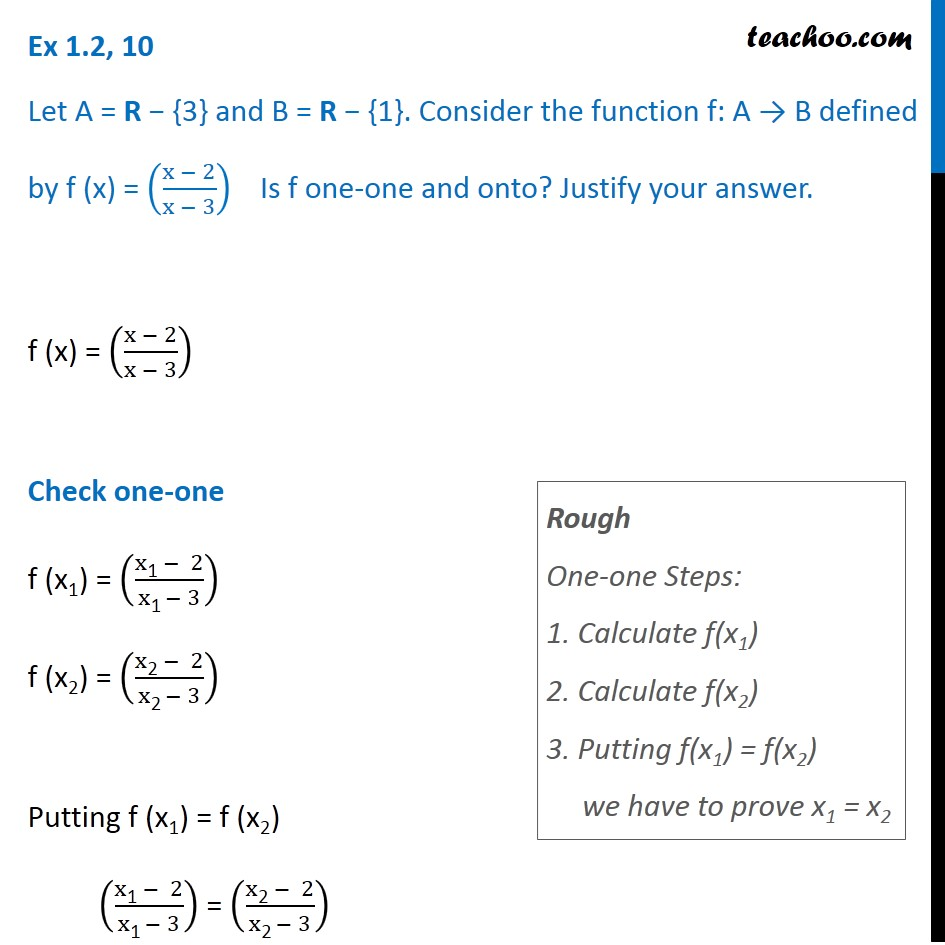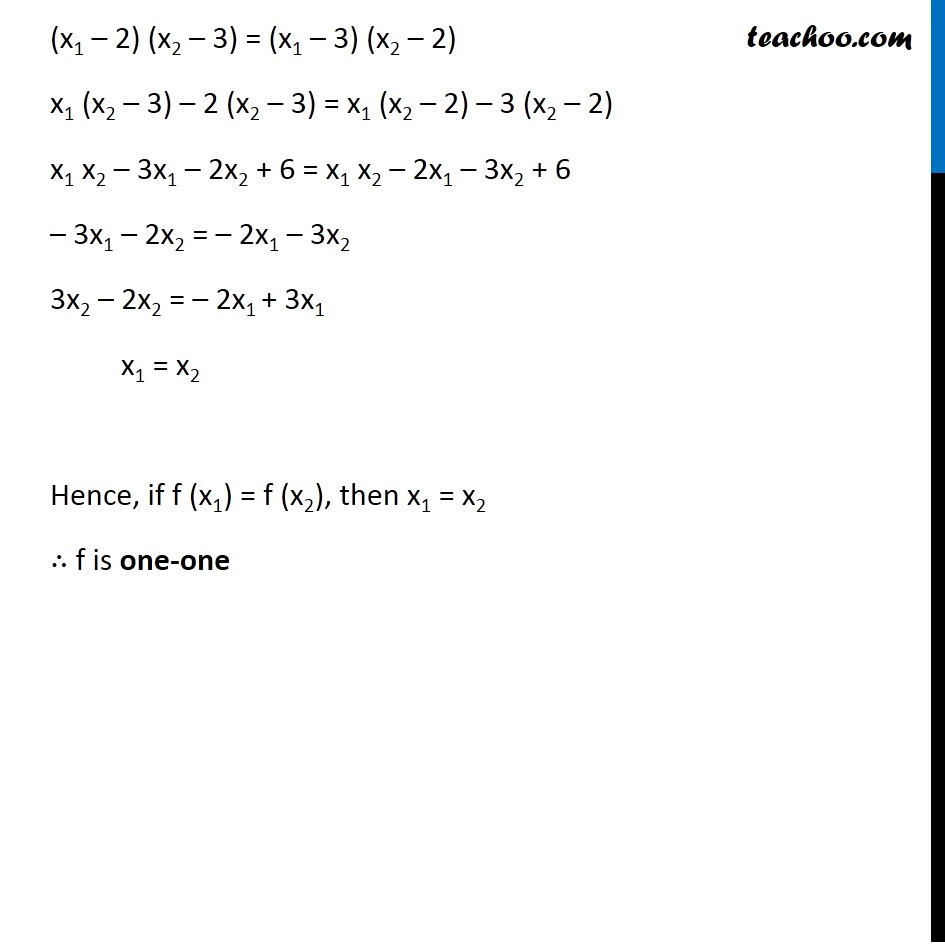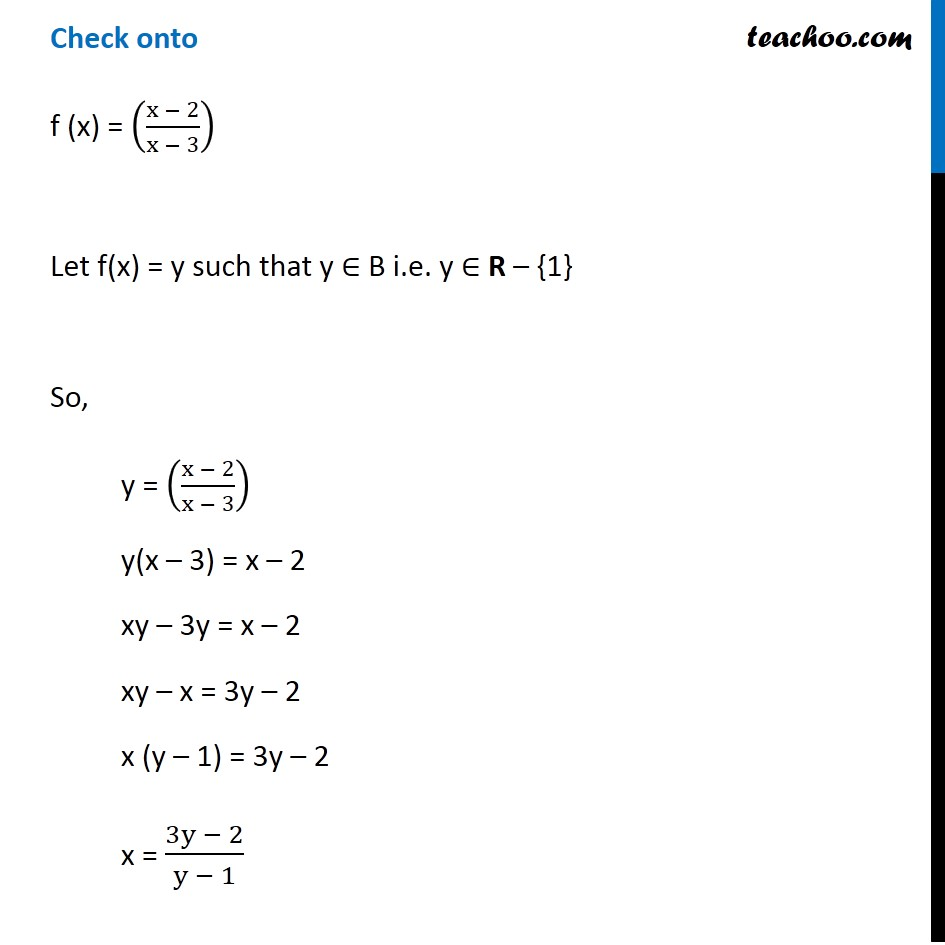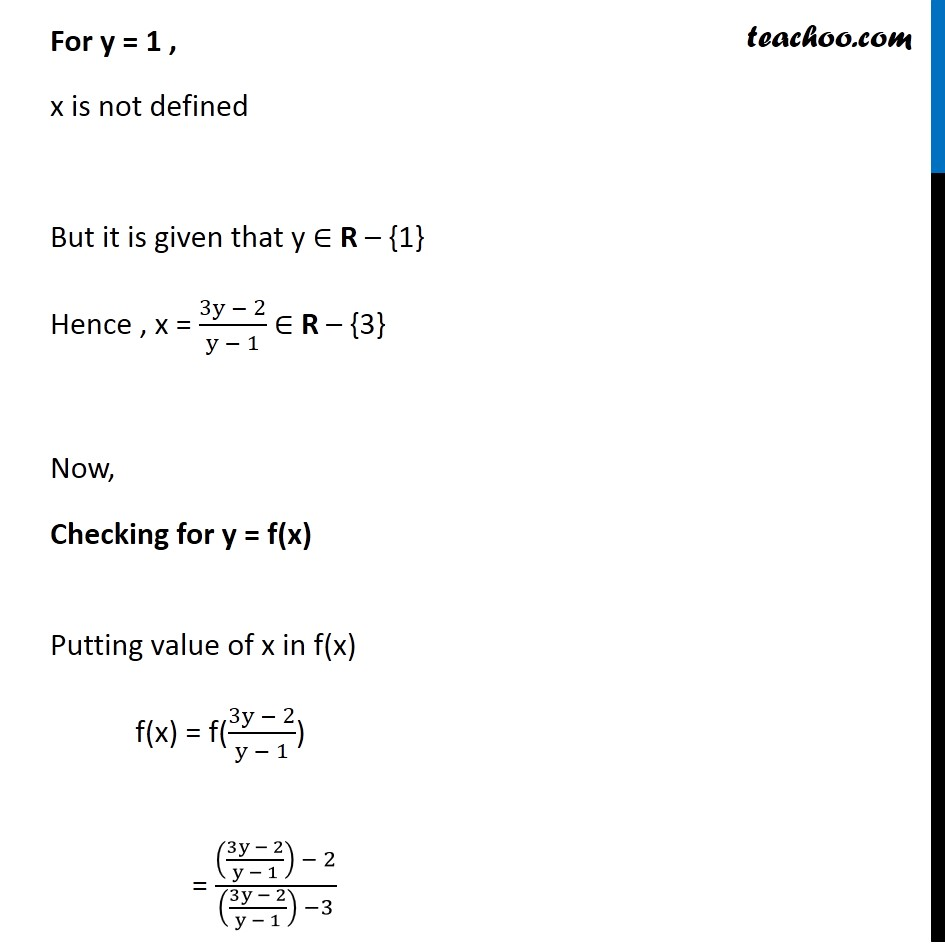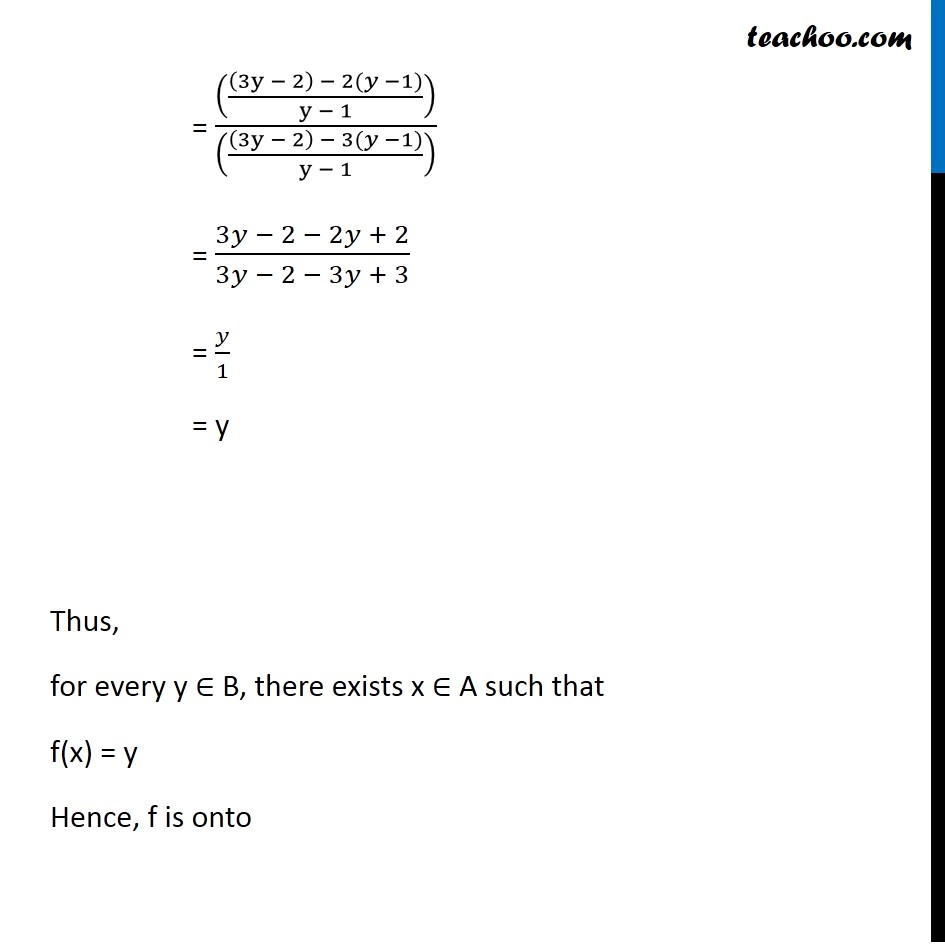Introducing your new favourite teacher - Teachoo Black, at only ₹83 per month

### Transcript

Ex 1.2, 10 Let A = R − {3} and B = R − {1}. Consider the function f: A → B defined by f (x) = ((x − 2)/(x − 3)) Is f one-one and onto? Justify your answer. f (x) = ((x − 2)/(x − 3)) Check one-one f (x1) = ((x"1 " − 2)/(x"1" − 3)) f (x2) = ((x"2 " − 2)/(x"2" − 3)) Putting f (x1) = f (x2) ((x"1 " − 2)/(x"1" − 3)) = ((x"2 " − 2)/(x"2" − 3)) Rough One-one Steps: 1. Calculate f(x1) 2. Calculate f(x2) 3. Putting f(x1) = f(x2) we have to prove x1 = x2 (x1 – 2) (x2 – 3) = (x1 – 3) (x2 – 2) x1 (x2 – 3) – 2 (x2 – 3) = x1 (x2 – 2) – 3 (x2 – 2) x1 x2 – 3x1 – 2x2 + 6 = x1 x2 – 2x1 – 3x2 + 6 – 3x1 – 2x2 = – 2x1 – 3x2 3x2 – 2x2 = – 2x1 + 3x1 x1 = x2 Hence, if f (x1) = f (x2), then x1 = x2 ∴ f is one-one Check onto f (x) = ((x − 2)/(x − 3)) Let f(x) = y such that y ∈ B i.e. y ∈ R – {1} So, y = ((x − 2)/(x − 3)) y(x – 3) = x – 2 xy – 3y = x – 2 xy – x = 3y – 2 x (y – 1) = 3y – 2 x = (3y − 2)/(y − 1) For y = 1 , x is not defined But it is given that y ∈ R – {1} Hence , x = (3y − 2)/(y − 1) ∈ R – {3} Now, Checking for y = f(x) Putting value of x in f(x) f(x) = f((3y − 2)/(y − 1)) = (((3y − 2)/(y − 1)) − 2)/(((3y − 2)/(y − 1)) −3) = ((((3y − 2) − 2(𝑦 −1))/(y − 1)))/((((3y − 2) − 3(𝑦 −1))/(y − 1)) ) = (3𝑦 − 2 − 2𝑦 + 2)/(3𝑦 − 2 − 3𝑦 + 3) = 𝑦/1 = y Thus, for every y ∈ B, there exists x ∈ A such that f(x) = y Hence, f is onto